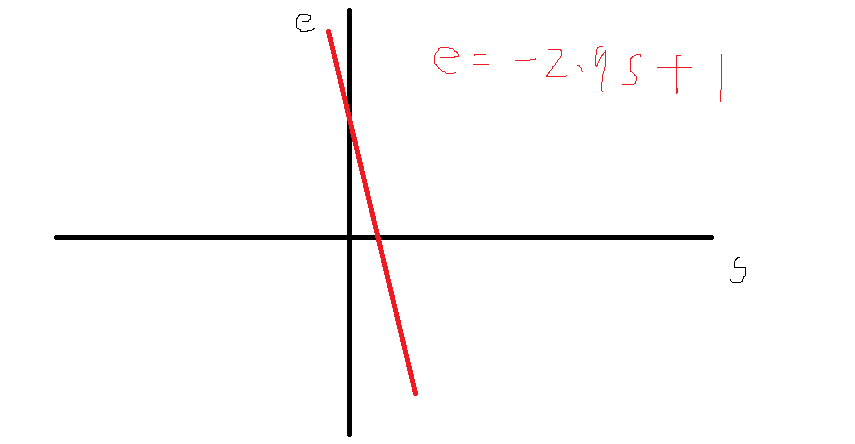•闪存
•博客
• 发言小组
• 投递新闻
• 提问博问
• 添加收藏
• 发布招聘
•文库

# 纯数学问题（因式分解，方程计算）

0悬赏园豆：20 [已解决问题] 解决于 2019-08-29 16:04`0.33,0.32,0.29,0.04,0.02`

`0.33a+0.32b+0.29c+0.04d+0.02e=1`
a,b,c,d,e之间的关系是
`a>b>c>d>e`
`a-b=b-c=c-d=d-e`

0gniing | 园豆：210 (菜鸟二级) | 2019-08-21 16:07

@gniing: 啊，谢谢指正。这些解可以用图形表示出来，画在直角坐标系里，落在第一象限里的数对都是解，所以有无穷多个解

gniing | 园豆：210 (菜鸟二级) | 2019-08-21 20:57

1.每个系数之间的间隔值设为s，得到下面4个等式

``````d=e+s
c=e+2s
b=e+3s
a=e+4s``````

2.系数调整之后的合计值仍为1，得到下面的等式

``0.33a+0.32b+0.29c+0.04d+0.02e=1``

3.将第1步中的等式全部用系数e和间隔值s表示，并带入第2步的等式中，得到下面的等式

``````0.33(e+4s) + 0.32(e+3s) + 0.29(e+2s) + 0.04(e+s) + 0.02e = 1
⇒0.33e+1.32s+0.32e+0.96s+0.29e+0.58s+0.04e+0.04s+0.02e=1
⇒e+2.9s=1``````

4.指定系数e为任意的正值，得到间隔值s，再由e和s求得其他系数的数值

``````d=0.7+0.1034=0.8034
c=0.7+2*0.1034=0.9068
b=0.7+3*0.1034=1.0102
a=0.7+4*0.1034=1.1136``````

@芽衣:0

zzhenxiang | 园豆：325 (菜鸟二级) | 2019-08-20 19:13
0

0

a=e+4k,
b=e+3k,
c=e+2k,
d=e+1k
e=e

e+2.81k=1

gniing | 园豆：210 (菜鸟二级) | 2019-08-21 09:50

c-d＝d-e，c=2d-e
c-d＝b-1, c=b+d
c-d＝a-b, c＝a-b+d
b-c = a-b, c =2b-a
b-c1 = d-e, c= b-d +e
0.33a+0.32b+0.29c+0.04d+0.02e=1

@亲吻冰封火焰: 题目说了，都是正数，楼主正解，看下面我写的

@亲吻冰封火焰: 不好意思，我后面那个系数算错了，楼下仁兄的第二个系数是对的，e>0是前提

您需要登录以后才能回答，未注册用户请先注册